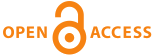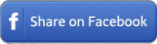Home|Journals|Articles by Year|Audio Abstracts RSS - TOCin any field in Title in Summary in Keywords in Authors in journals

 Original ResearchNatl J Physiol Pharm Pharmacol. 2022; 12(5): 695-698

#### Establishing and testing a multiple regression equation to predict body fat from height, weight, waist circumference, and hip circumference

Pawan Kumar Jha, Subhasis Mukherjee, Purab Kalyan Modak, Sharada Mayee Swain.

##### Abstract

Background: Body fat measurement requires a dedicated device and careful preparation of the subjects concerning food and water intake, exercise, precautions about alcohol intake, or any other condition that may change the hydration status of the subject. If simple anthropometric parameters can be used to estimate body fat that may help clinicians to know the body without measuring it.

Aim and Objectives: The aim of the study was to find a regression equation to estimate body fat from height, weight, waist circumference (WC), and hip circumference (HC).

Materials and Methods: First, we measured the height, weight, WC, HC, and body fat of 80 individuals with proper care for bioelectric impedance analysis. Then, this data were used to find multiple regression equation. Then, the equation was tested with 20 individuals where we measured the height, weight, WC, and HC and put those data into the equation to estimate body fat.

Results: The mean age of participants was 28.12 ± 3.45 years. The height, weight, WC, and HC all contributed statistically significantly to the prediction of body fat, F (4.75) = 31.17 P < 0.0001. The established regression equation was: Body fat = 40.134 - (height in cm × 0.271) + (weight in kg × 0.412) + (WC in cm × 0.052) + (HC in cm × 0.067). During the test of the equation, measured mean fat (36.79 ± 2.64%) was not statistically different (P = 0.75) from estimated body fat (36.6 ± 3.23%).

Conclusion: A multiple regression equation was formulated to estimate body fat from the height, weight, WC, and HC of an individual. This equation successfully estimated body fat from the anthropometric parameters. A further large-scale study is needed to find a more generalized estimation equation.

Key words: Anthropometry; Body Fat; Regression Equation; Body Mass Index; Obesity

 Share this ArticleOnline Article Submission
• ejmanager.com
• ojshosting.net